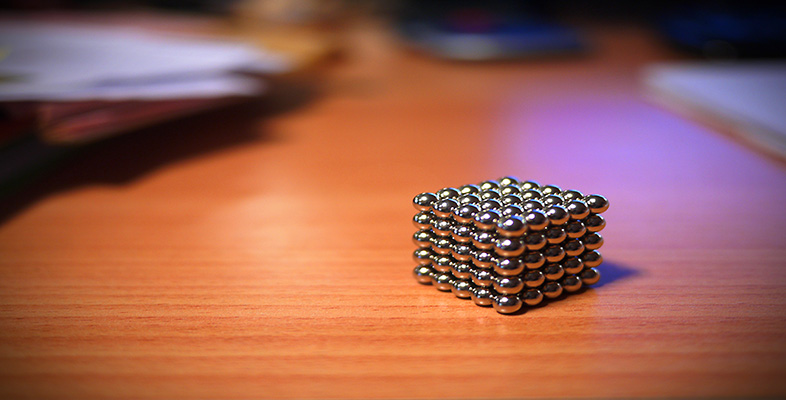Science, Maths & Technology

### Become an OU studentSquares, roots and powers

Start this free course now. Just create an account and sign in. Enrol and complete the course for a free statement of participation or digital badge if available.

# 3.1.1 Try some yourself

## Activity 33

Express each of the following numbers in scientific notation.

• (a) Light travels 9460 700 000 000 km in a year.

• (b) The average distance from the centre of the Earth to the centre of the Moon is 384 400 km.

• (c) Saturn is 1427 000 000 km from the Sun.

• (d) The mass of the Earth is 5976 000 000 000 000 000 000 000 kg.

• (e) The Mediterranean Sea has an area of 2504 000 km2.

• (f) Annapurna 1 is 8075 m high.

• (g) The diameter of the Sun is 1392 000 km. Use metres in your answer.

• (a) Light travels at 9.4607 × 1012 km in a year.

• (b) The distance from the centre of the Earth to the centre of the Moon is 3.844 × 105 km.

• (c) Saturn is 1.427 × 109 km from the Sun.

• (d) The mass of the Earth is 5.976 × 1024 kg.

• (e) The Mediterranean Sea has an area of 2.504 × 106 km2.

• (f) Annapurna 1 is 8.075 × 103 m high.

• (g) 1392 000 km is 1392 000 000 m (multiplying by 1000). In scientific notation this is

•   1.392 × 109 m.

• (Alternatively: 1392 000 km is 1.392 × 106 km = 1.392 × 106 × 103 m = 1.392 × 109 m.)

## Activity 34

The following numbers are given in scientific notation. Write each out as a single decimal number.

• (a) Ben Nevis is 1.343 × 103 m high.

• (b) Mercury is 5.8 × 107 km from the Sun.

• (c) The distance from the Earth to the nearest star is about 4.3 × 1013 km.

• (d) The Earth has a circumference of 4.0078 × 104 km at the equator.

• (e) The span of the Golden Gate Bridge is 1.28 × 103 m.

• (f) The Arctic Ocean has an area of 1.3986 × 107 km2.

• (a) Ben Nevis is 1343 m high.

• (b) Mercury is 58 000 000 km from the Sun.

• (c) The distance from the Earth to the nearest star is about 43 000 000 000 000 km.

• (d) The Earth has a circumference of 40 078 km at the equator.

• (e) The span of the Golden Gate Bridge is 1280 m.

• (f) The Arctic Ocean has an area of 13 986 000 km2.

## Activity 35

Express each of the following in scientific notation.

• (a) 0.53

• (b) 0.0075

• (c) 0.000 004

• (d) 0.000 020 01

• (a) 0.53 = 5.3 × 10−1

• (b) 0.0075 = 7.5 × 10−3

• (c) 0.000 004 = 4 × 10−6

• (d) 0.000 020 01 = 2.001 × 10−5

## Activity 36

Express each of the following in conventional decimal notation.

• (a) 2.03 × 10−4

• (b) 1.4 × 10−3

• (c) 5.67 × 10−5